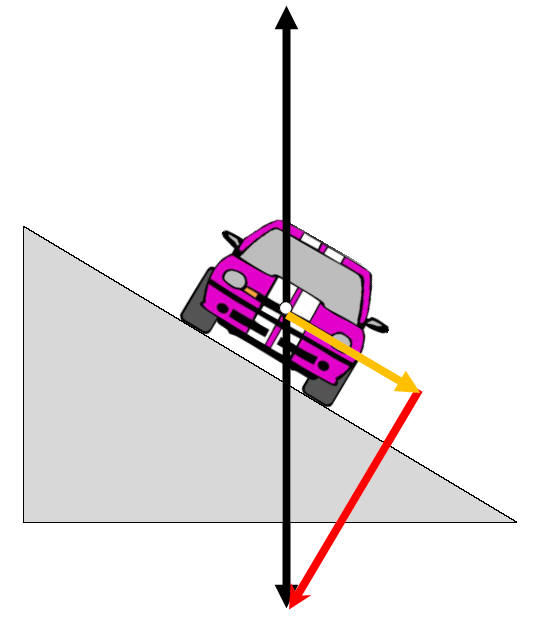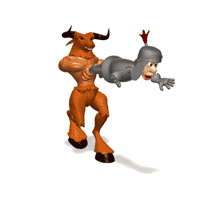Centripetal Force
Banked TrackedDraw the Weight ComponentsDraw AND LABEL the normal force

Hint: ∑Fy =  ?a) Find Fn Components

b) Label the FcExpress the weight mg of the car in terms of the normal force Fn and q

mg = Fncosq

Express the m, v and r in terms of Fn and q

Fc = mv2/r = Fnsinq

Combine the 2 previous equations into one

Fc = [v2]/gr = tanq

Rewrite an equation if the road has frictionHome